### AB/BA Crossover Design

Let "A" and "B" denote the two treatment values. Define the following notation: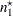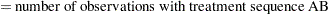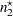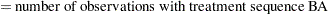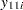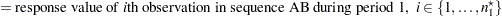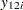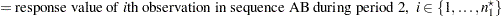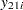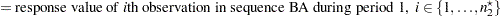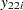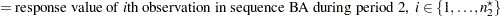So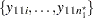and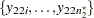are all observed at treatment level A, and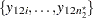and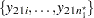are all observed at treatment level B.

Define the period difference for an observation as the difference between period 1 and period 2 response values: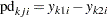for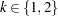and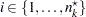. Similarly, the period ratio is the ratio between period 1 and period 2 response values: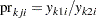The crossover difference for an observation is the difference between treatment A and treatment B response values: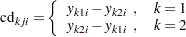Similarly, the crossover ratio is the ratio between treatment A and treatment B response values: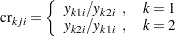In the absence of the IGNOREPERIOD option in the PROC TTEST statement, the data are split into two groups according to treatment sequence and analyzed as a two-independent-sample design. If DIST=NORMAL, then the analysis of the treatment effect is based on the half period differences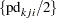, and the analysis for the period effect is based on the half crossover differences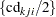. The computations for the normal difference analysis are the same as in the section Normal Difference (DIST=NORMAL TEST=DIFF) for the two-independent-sample design. The normal ratio analysis without the IGNOREPERIOD option is not supported for the AB/BA crossover design. If DIST=LOGNORMAL, then the analysis of the treatment effect is based on the square root of the period ratios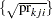, and the analysis for the period effect is based on the square root of the crossover ratios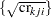. The computations are the same as in the section Lognormal Ratio (DIST=LOGNORMAL TEST=RATIO) for the two-independent-sample design.

If the IGNOREPERIOD option is specified, then the treatment effect is analyzed as a paired analysis on the (treatment A, treatment B) response value pairs, regardless of treatment sequence. So the set of pairs is taken to be the concatenation of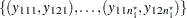and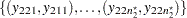. The computations are the same as in the section Paired Design.

See Senn (2002, Chapter 3) for a more detailed discussion of the AB/BA crossover design.